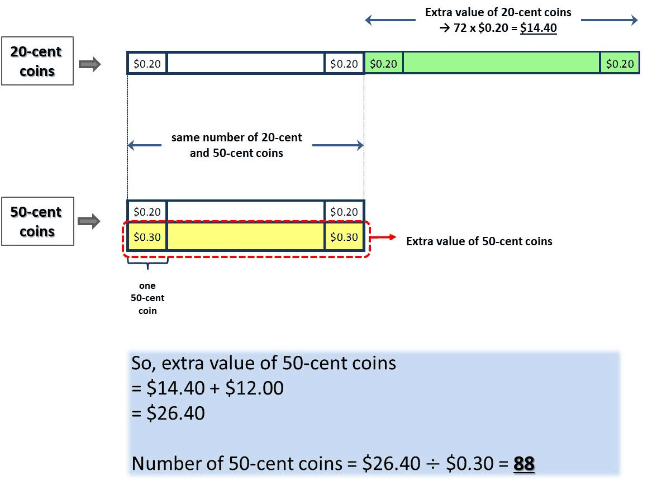# Question

Hi, we need help with this qn. Tq!

_____________

Ali puts some 50-cent and 20-cent coins in a box. There are 72 more 20-cent coins than 50-cent coins in the box. The total value of 50-cent coins is \$12 more than the total value of 20-cent coins. What is the number of 50-cent coins in the box?

2 Answers

# Answer

Number of coins in the box —
50-cent : 1unit
20-cent : 1 unit + 72
1 unit x 0.5 = (1 unit + 72) x 0.2 + 12
0.5 unit = 0.2 unit + 14.40 + 12 = 0.2 + 26.40
1 unit = 26.40/(0.5 – 0.2) = 88

Ans : 88 50-cent coins.

1 Reply 1 Like ✔Accepted Answer

Thank u!

1 Reply 0 Likes

U r most welcome!

0 Replies 0 Likescheers.

0 Replies 0 Likes